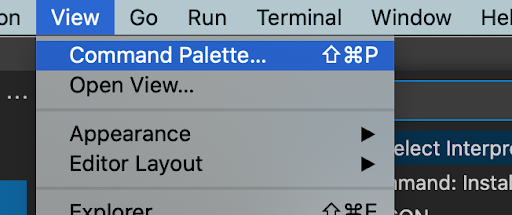Thursday, February 11, 2010

Convert currency to word in excel

There are plenty of online resources to convert Numeric currencies to Text- from \$200 to Dollar Two Hundred.
But this is applicable only ###,###,### (comma at every third number)format.  This is not suitable for India numbering system, because we follow ##,##,###(....22,22,222) format.

So I have create a excel function to over come this. You can use this function to to convert number up to 10,00,00,000(ten crores).

How to do it

Open Excel

Press Alt + F11

Copy paste the This Module there.

Exit from VBA window by pressing Alt + F11 again

In excel sheet, Insert Function,

Under user defined find ConvertCurrencyToEnglish

select it and provide the number as a parameter

thats all you are done
----------------------------------------------------------------------
----------------------------------------------------------------------

' ****  Author                 : Krishna S
' ****  Tittle                    : Converting Hindu Arabic Currency(Indian System) to Words
' ****  Description          : This utility converts currencies in Indian numbering system to words.
' ****  Limitations           : Converts only upto 10,00,00,000( Ten Crores)

Function ConvertCurrencyToEnglish(ByVal MyNumber)
Dim Temp
Dim Rupees, Paise
Dim DecimalPlace, Count
ReDim Place(9) As String
Place(2) = " Thousand "
Place(3) = " Lac "
Place(4) = " Core "
'   Place(5) = " Hundred Core "
' Convert MyNumber to a string, trimming extra spaces.
MyNumber = Trim(Str(MyNumber))
' Find decimal place.
DecimalPlace = InStr(MyNumber, ".")
' If we find decimal place...
If DecimalPlace > 0 Then
' Convert Paise
Temp = Left(Mid(MyNumber, DecimalPlace + 1) & "00", 2)
Paise = ConvertTens(Temp)
' Strip off Paise from remainder to convert.
MyNumber = Trim(Left(MyNumber, DecimalPlace - 1))
End If
Count = 1

Do While MyNumber <> ""
If Count = 1 Then

Temp = ConvertHundreds(Right(MyNumber, 3))

If Temp <> "" Then Rupees = Temp & Place(Count) & Rupees
If Len(MyNumber) > 3 Then
' Remove last 3 converted digits from MyNumber.
MyNumber = Left(MyNumber, Len(MyNumber) - 3)
Else
MyNumber = ""
End If
Count = Count + 1
Else
' Convert last 3 digits of MyNumber to English Rupees.
Temp = ConvertTens(Right(MyNumber, 2))

If Temp <> "" Then Rupees = Temp & Place(Count) & Rupees
If Len(MyNumber) > 3 Then
' Remove last 3 converted digits from MyNumber.
MyNumber = Left(MyNumber, Len(MyNumber) - 2)
Else
MyNumber = ""
End If
Count = Count + 1
End If
Loop
' Clean up Rupees.
Select Case Rupees
Case ""
Rupees = ""
Case "One"
Rupees = "One Rupee"
Case Else
Rupees = Rupees & " Rupees"
End Select
' Clean up Paise.
Select Case Paise
Case ""
Paise = ""
Case "One"
Paise = " And One Cent"
Case Else
Paise = " And " & Paise & " Paise"
End Select
ConvertCurrencyToEnglish = Rupees & Paise
End Function
Private Function ConvertHundreds(ByVal MyNumber)
Dim Result As String
' Exit if there is nothing to convert.
If Val(MyNumber) = 0 Then Exit Function
' Append leading zeros to number.
MyNumber = Right("000" & MyNumber, 3)
' Do we have a hundreds place digit to convert?
If Left(MyNumber, 1) <> "0" Then
Result = ConvertDigit(Left(MyNumber, 1)) & " Hundred "
End If
' Do we have a tens place digit to convert?
If Mid(MyNumber, 2, 1) <> "0" Then
Result = Result & ConvertTens(Mid(MyNumber, 2))
Else
' If not, then convert the ones place digit.
Result = Result & ConvertDigit(Mid(MyNumber, 3))
End If
ConvertHundreds = Trim(Result)
End Function
Private Function ConvertTens(ByVal MyTens)
Dim Result As String
' Is value between 10 and 19?
If Val(Left(MyTens, 1)) = 1 Then
Select Case Val(MyTens)
Case 10: Result = "Ten"
Case 11: Result = "Eleven"
Case 12: Result = "Twelve"
Case 13: Result = "Thirteen"
Case 14: Result = "Fourteen"
Case 15: Result = "Fifteen"
Case 16: Result = "Sixteen"
Case 17: Result = "Seventeen"
Case 18: Result = "Eighteen"
Case 19: Result = "Nineteen"
Case Else
End Select
Else
' .. otherwise it's between 20 and 99.
Select Case Val(Left(MyTens, 1))
Case 2: Result = "Twenty "
Case 3: Result = "Thirty "
Case 4: Result = "Forty "
Case 5: Result = "Fifty "
Case 6: Result = "Sixty "
Case 7: Result = "Seventy "
Case 8: Result = "Eighty "
Case 9: Result = "Ninety "
Case Else
End Select
' Convert ones place digit.
Result = Result & ConvertDigit(Right(MyTens, 1))
End If
ConvertTens = Result
End Function
Private Function ConvertDigit(ByVal MyDigit)
Select Case Val(MyDigit)
Case 1: ConvertDigit = "One"
Case 2: ConvertDigit = "Two"
Case 3: ConvertDigit = "Three"
Case 4: ConvertDigit = "Four"
Case 5: ConvertDigit = "Five"
Case 6: ConvertDigit = "Six"
Case 7: ConvertDigit = "Seven"
Case 8: ConvertDigit = "Eight"
Case 9: ConvertDigit = "Nine"
Case Else: ConvertDigit = ""
End Select
End Function
-----------------------------------------------------------

How to set Python3 as default interpreter for VS Code | or set any version as default interpreter.

Want to run your python code with a particular python version? And, make it the default version? Read on.. Make sure that the required versi...